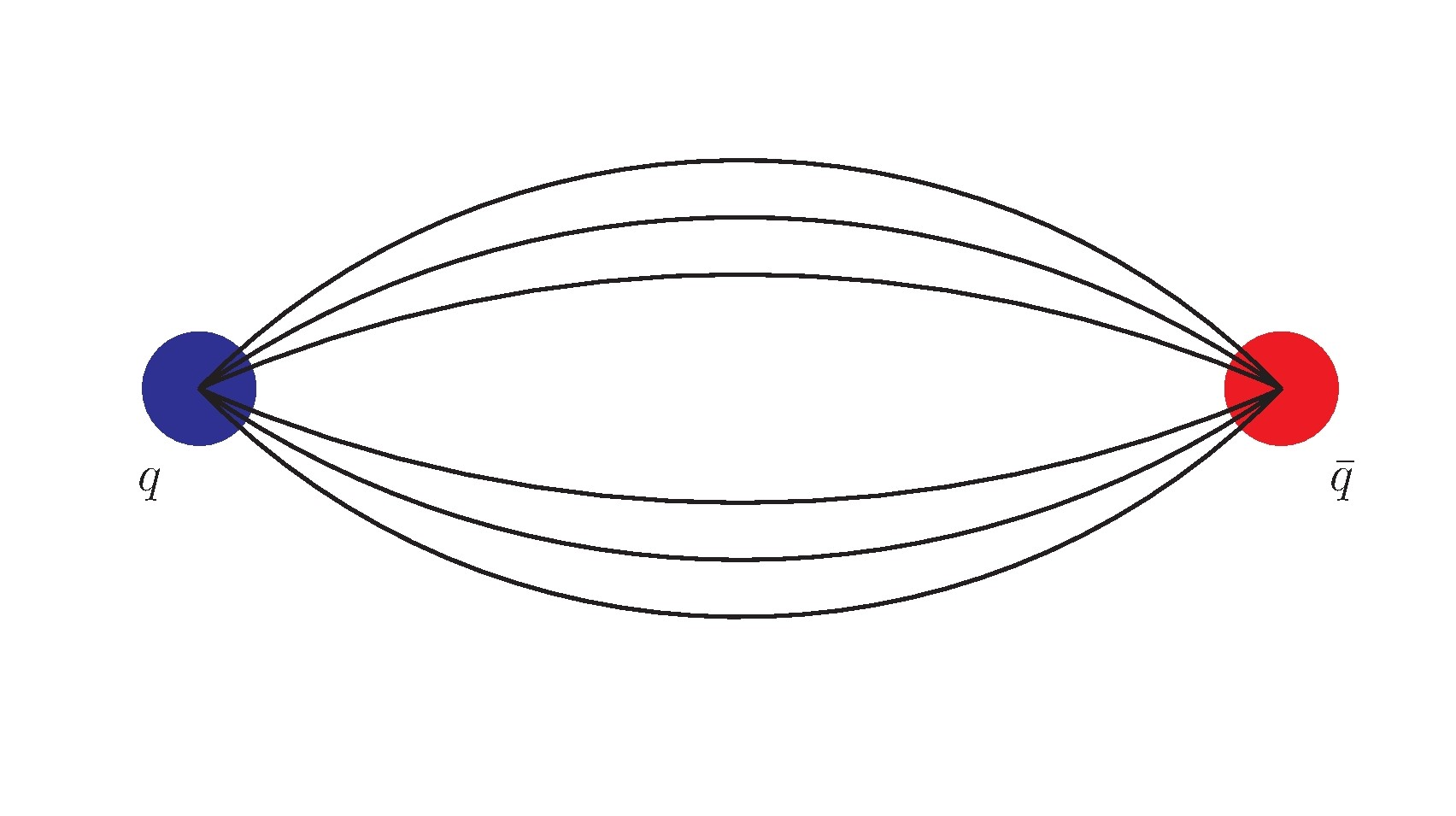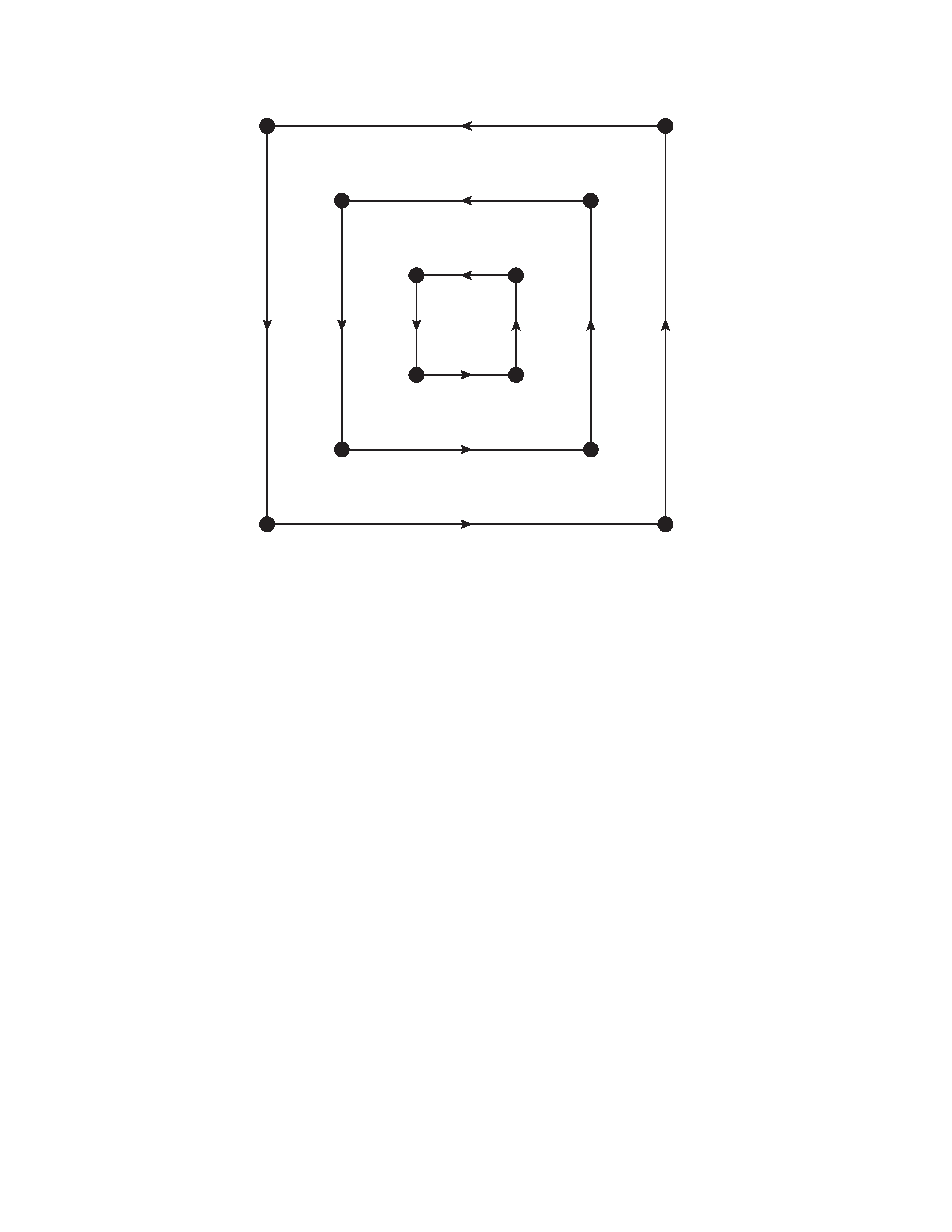2022-08-11

## Lattice action

We consider a $$U(1)$$ gauge theory in $$2+1$$ dimensions and regularize it on a asymmetric lattice (Degrand and DeTar (2006)):

$S = \frac{\beta}{\xi} \sum_{x} \sum_{\nu} \left[1 - \frac{1}{N_c} \operatorname{ReTr} (P_{0\nu}(x)) \right] + \beta \xi \sum_{x} \sum_{j<i} \left[1 - \frac{1}{N_c} \operatorname{ReTr} (P_{ij}(x)) \right]$ where $$P_{\mu \nu}$$ is the standard Wilson Plaquette (Gattringer and Lang (2009)): $P_{\mu \nu}(x) = U_\mu(x) U_{\nu}(x+\hat{\mu}) U_{\mu}^{\dagger}(x + \hat{\nu}) U_{\nu}^{\dagger}(x) %\quad (\mu,\nu=0,..,d-1) \quad .$ At the boundary we impose periodic boundary conditions. $$\beta=\frac{2 N_c}{g^2}$$ and $$\xi$$ is the bare anisotropy.

Due to its group structure, this theory is often referred as (here anisotropic) QED $$2+1$$ or QED$$_3$$.

It is relevant for some condensed matter systems (see e.g. Kosiński et al. (2012)).

It resembles QCD (in $$3+1$$ dimensions), showing:

• dynamical mass generation (Maris and Lee (2003))
• confinement (DeGrand (2019)).• It is studied with Monte Carlo techniques : $\mathcal{Z} = \int \mathcal{D} \phi e^{ - S[\phi]} = \int \mathcal{D} \phi e^{ - \sum_{x} \mathcal{L}[\phi](g_i^L)}$

software: https://github.com/urbach/su2

• There exist Hamiltonian formulations (Clemente et al. (2022)) suited to run on NISQ (Noisy Intermediate-Scale Quantum) devices in the near future. $U(t) = e^{-i H(g_i^H) t}$

We want to combine Lagrangian and Hamiltonian results

$$\to$$ required non-perturbative matching of the couplings $$g_i$$.

## Hamiltonian limit

A little background…

Lattice correlators are defined as:

$C_T(t) = \langle O_1(t) O_2(0) \rangle_T = \frac{\operatorname{Tr}[e^{- H(T-t) } O_1 e^{- H t} \, O_2]}{\operatorname{Tr} [e^{- H T}] } \\ = \frac{\sum_n \langle{n}| e^{- H(T-t) } O_1 e^{- H t} \, O_2 |{n}\rangle }{\sum_{n} \langle{n}| e^{- H T} |{n}\rangle}$

• We insert $$N$$ identity operators for each timeslice $$t_k = \epsilon k \, (\text{aka} \quad a_t k)$$: $\mathbb{1} = \sum_{m} \frac{1}{2 E_m} |m \rangle \langle m |$
• After some algebra we find:

$C_T(t) = \frac{\int \mathcal{D} \phi \, e^{-S_T[\phi]} O_1(t) O_2(0) }{\int \mathcal{D} \phi \, e^{-S[\phi]}}$ where $$S_T$$ is the discretized action (but with different lattice spacings in the time and space directions!).

• Now one says that in the limit $$a_t, a_s \to 0$$ spacetime symmetry is recovered. $$\implies$$ we define the path integral with $$a_t = a_s$$.
• The limit $$a_t \to 0 \, , \, a_s \neq 0$$ is the limit to the lattice Hamiltonian.

## Non perturbative matching

Steps:

• Calculation of $$n$$ observables $$O_i$$ in the two formalisms
• Lagrangian $$\to$$ Monte Carlo
• Hamiltonian $$\to$$ tensor network, quantum simulation, …
• In the action we introduce an anisotropy $$\xi$$ $$\implies$$ different temporal and spatial lattice spacings: $a_t \neq a_s$
• Find the limit $$a_t \to 0$$ of the $$O_i$$
• Find the couplings $$g^H_i$$ that match this limit of the Lagrangian

## Motivation

• Small $$\beta$$ region: harder determination of $$\xi_R$$ from the static potential

• Wilson flow renormalizes fields and the determination of $$\xi_R$$ from the Wilson flow is known to work in QCD (Borsányi et al. (2012))

Main idea

• Compute observables at fixed $$a_s$$:
• $\tau_0^2 E(\tau_0) = c$
• vary $$\xi$$ and find the $$\beta$$ s.t. $$a_s$$ is constant
• Find $$\xi_R = \xi(\tau_0)$$ for each ensemble
• Go to $$\xi_R \to 0$$
• Extrapolate to $$a_t \to 0$$ through $$\xi_R = a_t/a_s$$

## Matching with the Hamiltonian

Here we consider the pure gauge theory and want to match the 1st eigenvalue (mass gap). This is the lightest state of the theory (Loan and Ying (2006)): glueball $$0^{--}$$

Numerical studies in $$U(1)$$ (Athenodorou and Teper (2019)) and $$SU(N)$$ (Teper (1998)) theories find that the glueball $$0^{--}$$ is the lightest state.

Interpretation

In the continuum limit we expect a theory of free screened photons of mass $$m_D=m^{gs}_{0^{--}}$$ (Athenodorou and Teper (2019)):

$m^{ex1}_{0^{--}} = \frac{3}{2} m^{gs}_{0^{++}} = 3 m^{gs}_{0^{--}}$

## Glueball correlators

$\phi_i(x) = \operatorname{Tr} \left[ \prod_{k \in \mathcal{L}_i} U_{\mu_k}(x_k+\hat{e}_k) \right] \quad , \, x_1=x_n=x$

We use spatial square loops of size length $$r$$:

$U^{(r)}_{ij}(x) = \\ \prod_{k = 0}^r U_{i}(x+k\hat{e}_1) \prod_{k = 0}^r U_{j}(x+r\hat{e}_1+k\hat{e}_2) \times \\ \times \prod_{k = 0}^r U_{i}^{\dagger}(x+r\hat{e}_2+k\hat{e}_1) \prod_{k = 0}^r U_{j}^{\dagger}(x+k\hat{e}_1)$## $$PC$$ combination

Combination of $$\phi_i$$ with its transform under parity $$\phi^{(P)}$$ (Gattringer and Lang (2009)) and taking their real/imaginary part: $\phi_i^{PC} = \begin{pmatrix} \operatorname{Re} \\ \operatorname{Im} \end{pmatrix} \left[ \phi_i \pm \phi_i^{(P)} \right] \quad ,$

• Finally, we project to $$\vec{p}=\vec{0}$$: $\varphi_i^{PC}(t, \vec{0}) = \frac{1}{V} \sum_{\vec{x}} \phi_i^{PC}(t, \vec{x}) \\ = \frac{1}{L^2} \frac{2}{(d-1)(d-2)} \sum_{\vec{x}} \sum_{j<i} \operatorname{Re Tr} [ U_{ij}(t, \vec{x}) ]$

## Glueball correlation functions

The glueball correlators are build averaging over all possible timeslices:

$C_{r_i r_j}^{PC} (t) = \frac{1}{T} \sum_{\tau=1}^{T} \langle \varphi_{r_i}^{PC}(t+\tau) \varphi_{r_j}^{PC}(\tau) \rangle$

The large-time behavior is: $C_{r_i r_j}^{PC} (t) \to v_{r_i r_j} + A_{r_i r_j} (e^{-E_0^{PC} t} \pm e^{-E_0^{PC} (T-t)})$ where $$v_{r_i r_j}=\langle \varphi_{r_i}^{PC}(0) \varphi_j^{PC}(0) \rangle$$.

The VEV is subtracted exactly as $$C_{\text{sub}}(t) = C(t)-C(t+1)$$. Combining the $$C_{r_i r_j}$$ we can apply the GEVP.

(work in progress…)